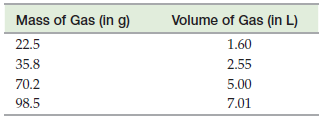×
Get Full Access to Introductory Chemistry - 5 Edition - Chapter 16 - Problem 54p
Get Full Access to Introductory Chemistry - 5 Edition - Chapter 16 - Problem 54p

×

What is the oxidation state of S in each ion?(a) SO42?(b) SO32?(c) HSO3?(d) HSO4?ISBN: 9780321910295 34

Solution for problem 54P Chapter 16

Introductory Chemistry | 5th Edition

• Textbook Solutions
• 2901 Step-by-step solutions solved by professors and subject experts
• Get 24/7 help from StudySoup virtual teaching assistantsIntroductory Chemistry | 5th Edition

4 5 1 320 Reviews
15
3
Problem 54P

A student prepares several samples of the same gas and measures their mass and volume. The results are tabulated as follows. Formulate a tentative law from the measurements.Step-by-Step Solution:

Step 1 of 2

From the given, tabulated values are as follows;Step 2 of 2

ISBN: 9780321910295

Unlock Textbook Solution